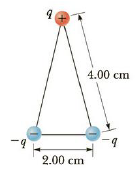Chapter 16, Problem 17P

Chapter
Section
Textbook Problem

The three charges in Figure P16.17 are at the vertices of an isosceles triangle. Let q = 7.00 nC and calculate the electric potential at the midpoint of the base.Figure P16.17

To determine
The potential at the midpoint.

Explanation

Formula to calculate the potential is,

V=keqr+keqr+keq+r+

• ke is the Coulomb constant.
• r and r+ is the distance between the charges q and q+ and the midpoint.

The between the q+ and the midpoint is,

r+=(4.00cm)2(1.00cm)2=3.87cm

Substitute 7.00 nC for q+ , -7.00 nC for q , 1.00 cm for r , 3.87 cm for r+ and 8.99×109 Nm2C2 for ke .

V=(8.99×109 Nm2C2){(7

Still sussing out bartleby?

Check out a sample textbook solution.

See a sample solution

The Solution to Your Study Problems

Bartleby provides explanations to thousands of textbook problems written by our experts, many with advanced degrees!

Get Started